# CAT 2020 Question Paper | DILR Slot 2

###### CAT Previous Year Paper | CAT DILR Questions | Question 11

The best questions to practice for CAT Exam are the actual CAT Question Papers. 2IIM offers you exactly that, in a student friendly format to take value from this. In CAT 2019 we saw some beautiful questions that laid emphasis on Learning ideas from basics and being able to comprehend more than remembering gazillion formulae and shortcuts. Original CAT Question paper is the best place to start off your CAT prep practice. This page provides exactly that. To check out about 1000 CAT Level questions with detailed video solutions for free, go here: CAT Question Bank

A chain of departmental stores has outlets in Delhi, Mumbai, Bengaluru and Kolkata. The sales are categorized by its three departments – ‘Apparel’, ‘Electronics’, and ‘HomeDecor’. An Accountant has been asked to prepare a summary of the 2018 and 2019 sales amounts for an internal report. He has collated partial information and prepared the following table.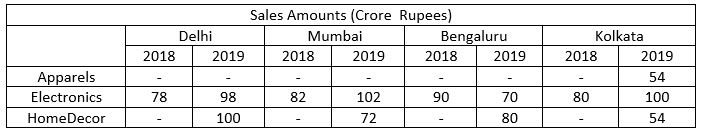The following additional information is known.
1. The sales amounts in the Apparel departments were the same for Delhi and Kolkata in 2018.
2. The sales amounts in the Apparel departments were the same for Mumbai and Bengaluru in 2018. This sales amount matched the sales amount in the Apparel department for Delhi in 2019.
3. The sales amounts in the HomeDecor departments were the same for Mumbai and Kolkata in 2018.
4. The sum of the sales amounts of four Electronics departments increased by the same amount as the sum of the sales amounts of four Apparel departments from 2018 to 2019.
5. The total sales amounts of the four HomeDecor departments increased by Rs 70 Crores from 2018 to 2019.
6. The sales amounts in the HomeDecor departments of Delhi and Bengaluru each increased by Rs 20 Crores from 2018 to 2019.
7. The sales amounts in the Apparel departments of Delhi and Bengaluru each increased by the same amount in 2019 from 2018. The sales amounts in the Apparel departments of Mumbai and Kolkata also each increased by the same amount in 2019 from 2018.
8. The sales amounts in the Apparel departments of Delhi, Kolkata and Bengaluru in 2019 followed an Arithmetic Progression.

Question 11 : Among all the 12 departments (i.e., the 3 departments in each of the 4 cities), what was the maximum percentage increase in sales amount from 2018 to 2019?

1. 75
2. 50
3. 25
4. 28

## Best CAT Coaching in Chennai

#### CAT Coaching in Chennai - CAT 2021Online Batches Available Now!

Before we begin solving, let's try to apply the easiest points to the table to see how much info we can get (Key is to apply the easiest looking rules first so that we can get as many missing numbers as possible, not a good idea to apply the rules in the order that they are given)
For now, let us apply points 1, 2, 3, 6 and see how the table looks: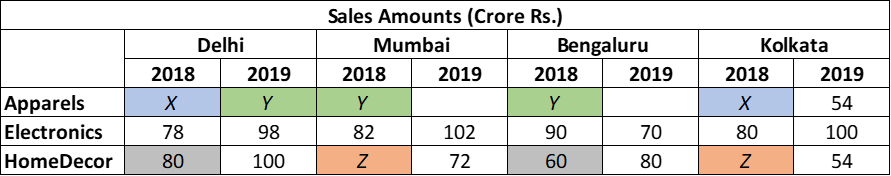Now let's apply point 5 so that we can get the missing value Z
(100+72+80+54) - (80 + Z + 60 + Z) = 70
306 - 140 - 2(Z) = 70
166 - 2(Z) = 70
2(Z) = 96
Z = 48

This is how the table looks as of now: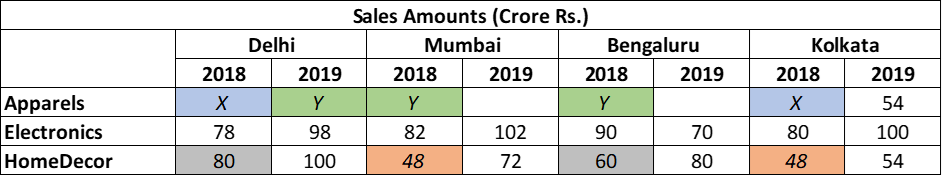Let's continue to solve the table, let's apply point 7 now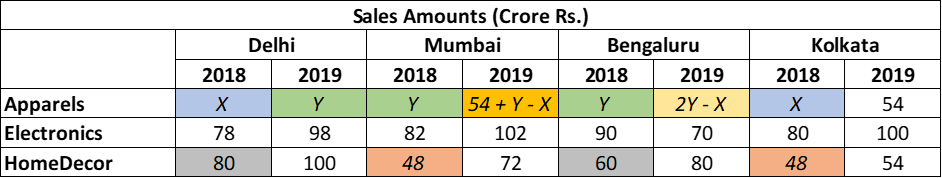Let's apply point 8.
Since Sales for Delhi, Kolkata and Bangalore in 2019 form an AP, the following must be true:

K (2019) - D (2019) = B (2019) - K (2019)
54 - Y = (2Y - X) - 54
108 = 3Y - X …........................(Equation 1)

Finally, let's apply point 4.

(98 + 102 + 70 + 100) - (78 + 82 + 90 + 80) = (Y + 54 + Y - X + 2Y - X + 54) - (X + Y + Y + X)
370 - 330 = (108 + 4Y - 2X) - (2Y + 2X)
40 = 108 + 2Y - 4X
4X - 2Y = 68…........................(Equation 2)

Solve equations 1 and 2

4X - 2Y= 68
12Y - 4X = 432
10Y = 500

Y = 50
X = 42

The finished table looks like this: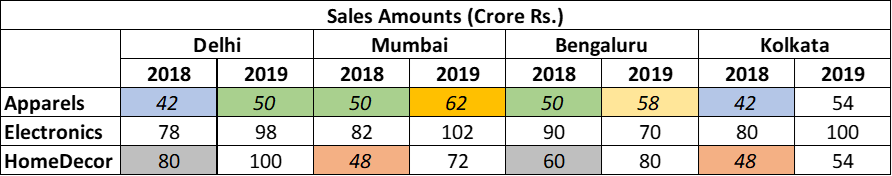A quick glance at the table can tell us that the % will be highest where the base is low and the increase is high. A strong candidate is Mumbai in HomeDecor from 2018-19 (50% increase), no other cell seems to fit the bill

The question is "Among all the 12 departments (i.e., the 3 departments in each of the 4 cities), what was the maximum percentage increase in sales amount from 2018 to 2019?"

##### Hence, the answer is, "50"

###### CAT Coaching in ChennaiCAT 2021Enroll at 49,000/- 44,000/-

Online Classroom Batches Starting Now!

###### Best CAT Coaching in Chennai Introductory offer of 5000/-

Attend a Demo Class

##### Where is 2IIM located?

2IIM Online CAT Coaching
A Fermat Education Initiative,
58/16, Indira Gandhi Street,
Kaveri Rangan Nagar, Saligramam, Chennai 600 093

##### How to reach 2IIM?

Phone: (91) 44 4505 8484
Mobile: (91) 99626 48484
WhatsApp: WhatsApp Now
Email: prep@2iim.com